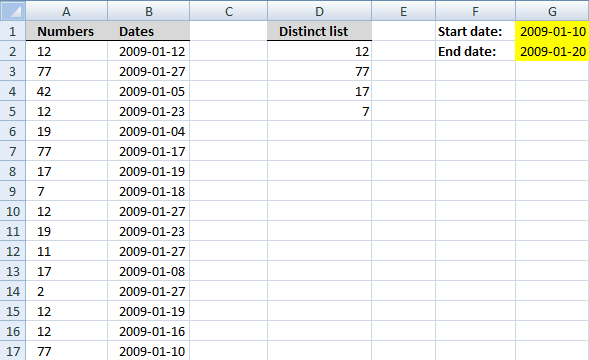Author: Oscar Cronquist Article last updated on January 03, 2019Question: I have a large list of dates and other adjacent values. I want to create a distinct list from the large list based on criteria, only values within a specific date interval.

The date range is specified in cell G1 and G2, see image above.

Array formula in cell D2:

=IFERROR(INDEX(\$A\$2:\$A\$17, MATCH(0,COUNTIF(\$D\$1:D1, IF((\$G\$2>=\$B\$2:\$B\$17)*(\$G\$1<=\$B\$2:\$B\$17), \$A\$2:\$A\$17, \$D\$1)), 0)), "")

To enter an array formula, type the formula in a cell then press and hold CTRL + SHIFT simultaneously, now press Enter once. Release all keys.

The formula bar now shows the formula with a beginning and ending curly bracket telling you that you entered the formula successfully. Don't enter the curly brackets yourself.

### Explaining the formula in cell D2

#### Step 1 - Filter numbers in date range

IF((\$G\$2>=\$B\$2:\$B\$17)*(\$G\$1<=\$B\$2:\$B\$17),\$A\$2:\$A\$17,\$D\$1)

becomes

IF((39833>={39825; 39840; 39818; 39836; 39817; 39830; 39832; 39831; 39840; 39836; 39840; 39821; 39840; 39832; 39829; 39823})*(39823<={39825; 39840; 39818; 39836; 39817; 39830; 39832; 39831; 39840; 39836; 39840; 39821; 39840; 39832; 39829; 39823}),{12; 77; 42; 12; 19; 77; 17; 7; 12; 19; 11; 17; 2; 12; 12; 77},"Unique distinct list")

and returns

{12; "Unique distinct list"; "Unique distinct list"; "Unique distinct list"; "Unique distinct list"; 77; 17; 7; "Unique distinct list"; "Unique distinct list"; "Unique distinct list"; "Unique distinct list"; "Unique distinct list"; 12; 12; 77}

How to use the IF function

Checks if a logical expression is met. Returns a specific value if TRUE and another specific value if FALSE.

#### Step 2 - Check numbers already displayed

COUNTIF(\$D\$1:D1,IF((\$G\$2>=\$B\$2:\$B\$17)*(\$G\$1<=\$B\$2:\$B\$17),\$A\$2:\$A\$17,\$D\$1))

becomes

COUNTIF(\$D\$1:D1,{12; "Unique distinct list"; "Unique distinct list"; "Unique distinct list"; "Unique distinct list"; 77; 17; 7; "Unique distinct list"; "Unique distinct list"; "Unique distinct list"; "Unique distinct list"; "Unique distinct list"; 12; 12; 77})

and returns

{0; 1; 1; 1; 1; 0; 0; 0; 1; 1; 1; 1; 1; 0; 0; 0}

How to use the COUNTIF function

Counts the number of cells that meet a specific condition.

Step 3 - Find position of next unique distinct number

MATCH(0,COUNTIF(\$D\$1:D1,IF((\$G\$2>=\$B\$2:\$B\$17)*(\$G\$1<=\$B\$2:\$B\$17),\$A\$2:\$A\$17,\$D\$1)),0)

becomes

MATCH(0,{0; 1; 1; 1; 1; 0; 0; 0; 1; 1; 1; 1; 1; 0; 0; 0},0)

and returns 1.

How to use the MATCH function

Identify the position of a value in an array.

#### Step 4 - Return number

INDEX(\$A\$2:\$A\$17,MATCH(0,COUNTIF(\$D\$1:D1,IF((\$G\$2>=\$B\$2:\$B\$17)*(\$G\$1<=\$B\$2:\$B\$17),\$A\$2:\$A\$17,\$D\$1)),0))

becomes

INDEX(\$A\$2:\$A\$17,1)

becomes

INDEX({12; 77; 42; 12; 19; 77; 17; 7; 12; 19; 11; 17; 2; 12; 12; 77},1)

and returns 12 in cell D2.

How to use the INDEX function

Gets a value in a specific cell range based on a row and column number.